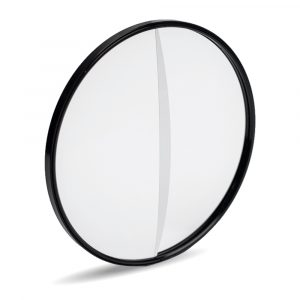## Diopters

Diopter or ‘dioptre’  from the Greek di optos (through + visible) has a number of meanings in optics, including being a unit for the refractive power of a lens.

##### Full Field Diopters.

In the world of filters, close up diopters are the equivalent of a magnifying glass for your lens.  So among other things, they are a simple and visually striking way of producing macro-style images, as these examples from Tiffen demonstate.

Diopters do this because they have a curvature which will reduce the focal length of the lens you are using, enabling a prime lens to focus on items closer than its normal focal length. This is particularly useful for anamorphic lenses which are much harder to close focus.

The increasing power of the diopter – ie the greater its curvature – the shorter the adjusted focal length of your lens will become, according to a mathematical equation.

The equation to provide you with your new focus is as follows:

New Close Focus Distance (in metres) = old close focus distance  divided by (Diopter strength x old close focus distance ) +1)

So if you have a lens with a close focus of a half meter and a strength 4 Diopter filter then your equation is as follows

New Close Focus Distance = 0.5 Original / ((4 Diopterx 0.5 Original) +1 constant) ie 0.5 / 3 = 0.167

Let’s run through that – our diopter strength is 4, our original close distance is 0.5 metres – so four multiplied by the half – that gives us 2.

We then add our constant of 1 to the 2,  giving us 3 – which means we divide the total original focal length of 0.5m by 3. That gives us a new close distance of 0.167m.

(NB – to use cm instead of metres, your constant becomes 100, instead of 1. So the calculation would be 50 / ((4 x 50 )+100) = 50 / (200 + 100) = 0.167 – note that your answer will still be in metres).

Full field diopters then provide a quick and easy alternative to a macro lens which can give instant close ups and spectacular and slightly etherial images – particularly useful for nature photography.

##### Split diopters.As the title suggest, split diopters only magnify on one side. The purpose of this is to allow both foreground and background to be in focus at the same time.

Split-Field Diopters are a standard diopter element, precision-cut in half and mounted into a ring. Frame your subject, focus on the distant background and then move the camera slightly to obtain close focus on the foreground.

All you then need to do is position the slightly out-of-focus line of the cut edge of the glass in an area it won‘t be noticed and closing the iris can help reduce the width of this line.

And the effect – as these examples from Schneider show – is spectacular .

For more information on the diopters we stock and to make an enquiry, check out our full range here.

And you can work out the strength of diopter you need here, with our handy calculator.